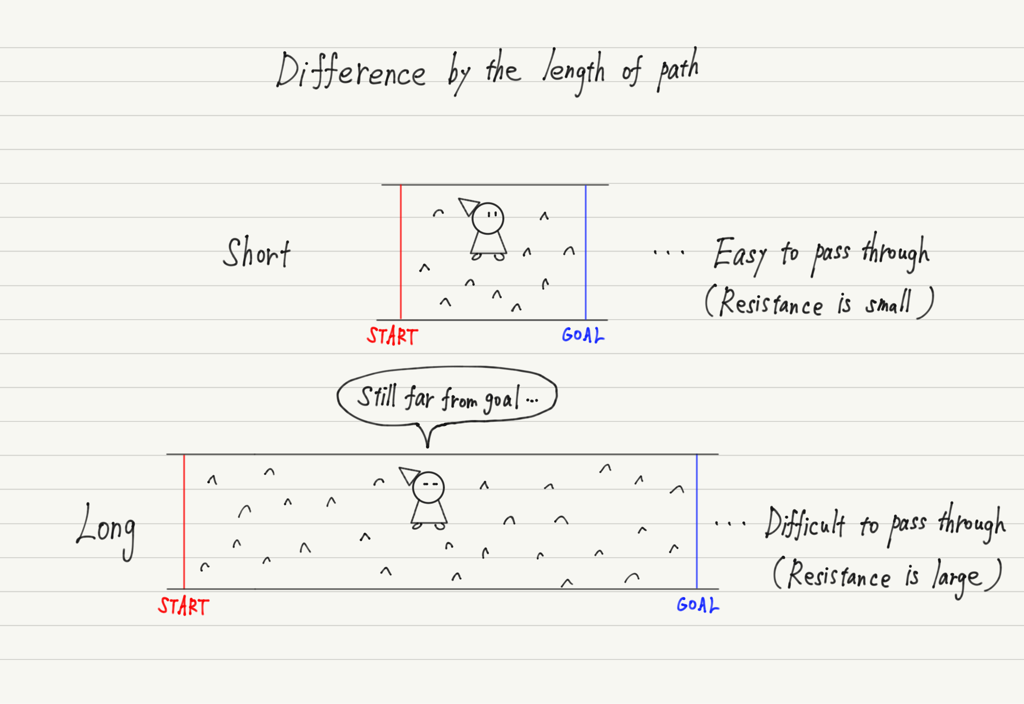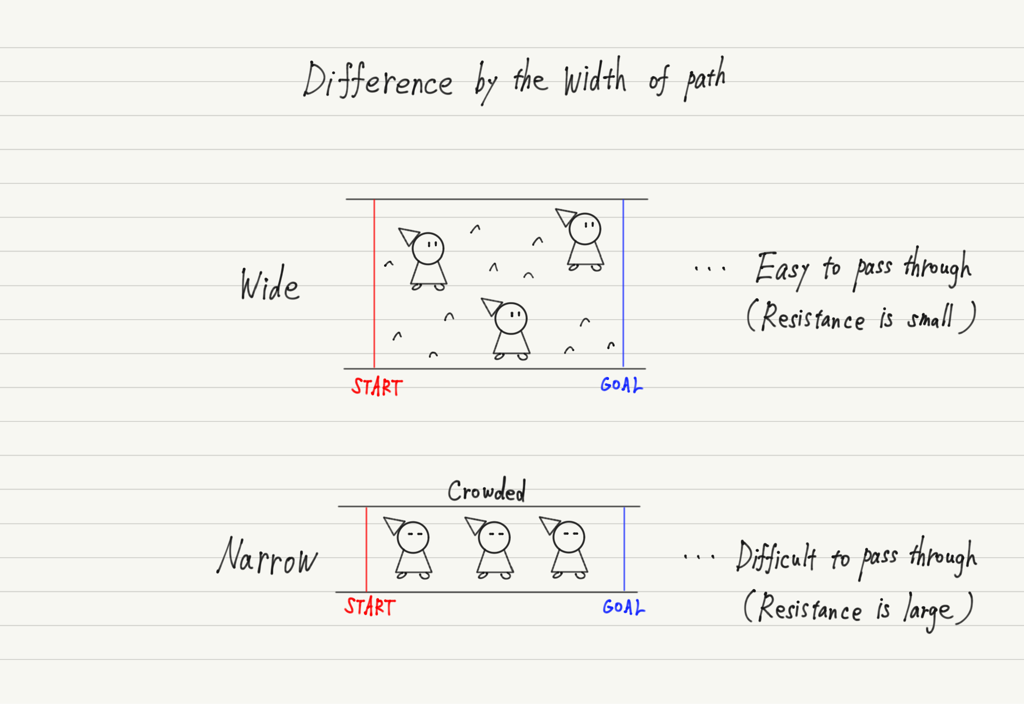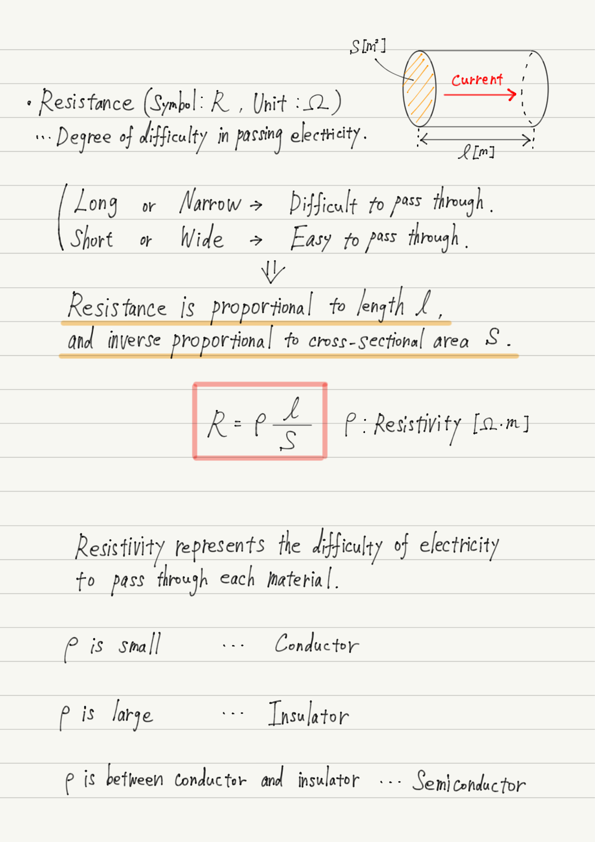# Resistance and Resistivity

(This article was translated from Japanese to English using DeepL.)

Do you know Ohm’s law?
Ohm’s law is expressed by the formula “Voltage ＝ Resistance ✕ Electric Current”.

However, to solve electric circuit problems, it is not enough to memorize this formula; it is necessary to understand what this formula represents.
Before we get to Ohm’s law, we need to deepen our understanding of Voltage, Resistance, and Electric Current respectively. This lecture will focus on Resistance.

## Image of Resistance

Conductors allow current to pass through them, but there is a certain degree of “Difficulty” to pass through them. The resistance is a quantification of the “Difficulty”. The unit is Ω.

What determines the value of resistance?
Many people expect resistance to be determined by material, but that is not a perfect answer. This is because the same material can be used to create different resistances.

To understand this, imagine “Resistance = Bumpy path”. In the following, we will use this image of a bumpy path to illustrate.

## Resistor Shape and Resistance

What determines the difficulty of passing on this bumpy path?
The answers are: 1) Length of the path, 2) Width of the path, and 3) Degree of bumpiness.

As for 1), you can imagine that the longer the length is, the harder it is to pass through, even if the degree of bumpiness is the same.If the length of the path doubles, the time it takes to pass through doubles (if the speed is constant).
This means that the resistance is proportional to the length of the path.

Next is 2), which should also be easy to visualize. It is only natural that the wider the path, the easier it is to pass through.In other words, resistance is inverse proportional to the width of the path.
（Since the resistance is actually cylindrical, the path width refers to the cross-sectional area of the cylinder.）

Finally, 3). You have understood that the length and width of a road can change the difficulty of passing through it.
However, even if the length and width are the same, it does not necessarily mean that the difficulty of passing through is the same. This is because the degree of bumpiness may vary.

The “difference in the degree of bumpiness” when compared with the same length and width represents the “difficulty of passing electric current for each material”. This is called the resistivity of the material.

Depending on the value of resistivity, substances are classified as conductors or Insulators.
As a supplement, there are materials in the world with resistivity that are smaller than conductors but larger than insulators. Such materials are called semiconductors and are important to modern technology.

## Summary of this lecture## Next Time

The next lecture will be on electric current.

https://www.yukimura-physics.com/entry/elemag-f05

error: Content is protected !!